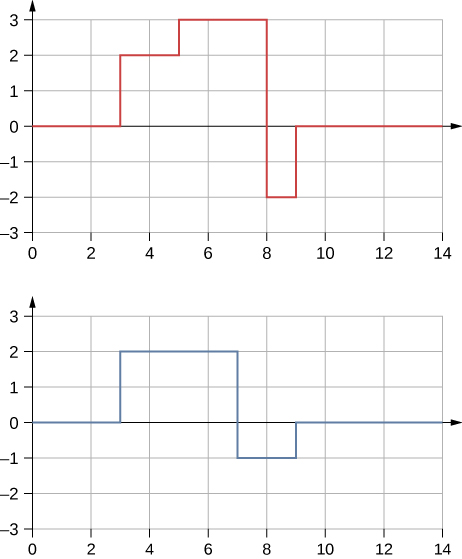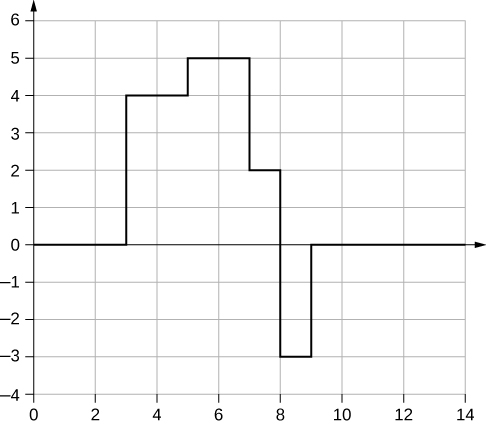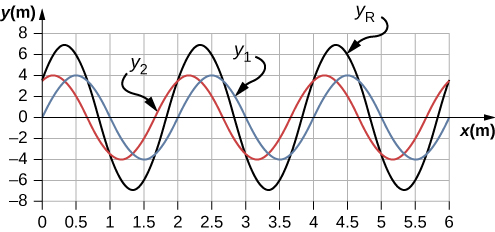16.5 Interference of waves  (Page 6/12)

 Page 6 / 12

A long, tight spring is held by two students, one student holding each end. Each student gives the end a flip sending one wavelength of a sinusoidal wave down the spring in opposite directions. When the waves meet in the middle, what does the wave look like?

Many of the topics discussed in this chapter are useful beyond the topics of mechanical waves. It is hard to conceive of a mechanical wave with sharp corners, but you could encounter such a wave form in your digital electronics class, as shown below. This could be a signal from a device known as an analog to digital converter, in which a continuous voltage signal is converted into a discrete signal or a digital recording of sound. What is the result of the superposition of the two signals?A string of a constant linear mass density is held taut by two students, each holding one end. The tension in the string is constant. The students each send waves down the string by wiggling the string. (a) Is it possible for the waves to have different wave speeds? (b) Is it possible for the waves to have different frequencies? (c) Is it possible for the waves to have different wavelengths?

Problems

Consider two sinusoidal waves traveling along a string, modeled as ${y}_{1}\left(x,t\right)=0.3\phantom{\rule{0.2em}{0ex}}\text{m}\phantom{\rule{0.2em}{0ex}}\text{sin}\left(4\phantom{\rule{0.2em}{0ex}}{\text{m}}^{-1}x+3\phantom{\rule{0.2em}{0ex}}{\text{s}}^{-1}t\right)$ and ${y}_{2}\left(x,t\right)=0.6\phantom{\rule{0.2em}{0ex}}\text{m}\phantom{\rule{0.2em}{0ex}}\text{sin}\left(8\phantom{\rule{0.2em}{0ex}}{\text{m}}^{-1}x-6\phantom{\rule{0.2em}{0ex}}{\text{s}}^{-1}t\right).$ What is the height of the resultant wave formed by the interference of the two waves at the position $x=0.5\phantom{\rule{0.2em}{0ex}}\text{m}$ at time $t=0.2\phantom{\rule{0.2em}{0ex}}\text{s?}$

Consider two sinusoidal sine waves traveling along a string, modeled as ${y}_{1}\left(x,t\right)=0.3\phantom{\rule{0.2em}{0ex}}\text{m}\phantom{\rule{0.2em}{0ex}}\text{sin}\left(4\phantom{\rule{0.2em}{0ex}}{\text{m}}^{-1}x+3\phantom{\rule{0.2em}{0ex}}{\text{s}}^{-1}t+\frac{\pi }{3}\right)$ and ${y}_{2}\left(x,t\right)=0.6\phantom{\rule{0.2em}{0ex}}\text{m}\phantom{\rule{0.2em}{0ex}}\text{sin}\left(8\phantom{\rule{0.2em}{0ex}}{\text{m}}^{-1}x-6\phantom{\rule{0.2em}{0ex}}{\text{s}}^{-1}t\right).$ What is the height of the resultant wave formed by the interference of the two waves at the position $x=1.0\phantom{\rule{0.2em}{0ex}}\text{m}$ at time $t=3.0\phantom{\rule{0.2em}{0ex}}\text{s?}$

$y\left(x,t\right)=0.76\phantom{\rule{0.2em}{0ex}}\text{m}$

Consider two sinusoidal sine waves traveling along a string, modeled as ${y}_{1}\left(x,t\right)=0.3\phantom{\rule{0.2em}{0ex}}\text{m}\phantom{\rule{0.2em}{0ex}}\text{sin}\left(4\phantom{\rule{0.2em}{0ex}}{\text{m}}^{-1}x-3\phantom{\rule{0.2em}{0ex}}{\text{s}}^{-1}t\right)$ and ${y}_{2}\left(x,t\right)=0.3\phantom{\rule{0.2em}{0ex}}\text{m}\phantom{\rule{0.2em}{0ex}}\text{sin}\left(4\phantom{\rule{0.2em}{0ex}}{\text{m}}^{-1}x+3\phantom{\rule{0.2em}{0ex}}{\text{s}}^{-1}t\right).$ What is the wave function of the resulting wave? [ Hint: Use the trig identity $\text{sin}\left(u±v\right)=\text{sin}\phantom{\rule{0.2em}{0ex}}u\phantom{\rule{0.2em}{0ex}}\text{cos}\phantom{\rule{0.2em}{0ex}}v±\phantom{\rule{0.2em}{0ex}}\text{cos}\phantom{\rule{0.2em}{0ex}}u\phantom{\rule{0.2em}{0ex}}\text{sin}\phantom{\rule{0.2em}{0ex}}v$

Two sinusoidal waves are moving through a medium in the same direction, both having amplitudes of 3.00 cm, a wavelength of 5.20 m, and a period of 6.52 s, but one has a phase shift of an angle $\varphi$ . What is the phase shift if the resultant wave has an amplitude of 5.00 cm? [ Hint: Use the trig identity $\text{sin}\phantom{\rule{0.2em}{0ex}}u+\text{sin}\phantom{\rule{0.2em}{0ex}}v=2\phantom{\rule{0.2em}{0ex}}\text{sin}\left(\frac{u+v}{2}\right)\text{cos}\left(\frac{u-v}{2}\right)$

${A}_{R}=2A\phantom{\rule{0.2em}{0ex}}\text{cos}\left(\frac{\varphi }{2}\right),\phantom{\rule{0.2em}{0ex}}\varphi =1.17\phantom{\rule{0.2em}{0ex}}\text{rad}$

Two sinusoidal waves are moving through a medium in the positive x -direction, both having amplitudes of 6.00 cm, a wavelength of 4.3 m, and a period of 6.00 s, but one has a phase shift of an angle $\varphi =0.50\phantom{\rule{0.2em}{0ex}}\text{rad}.$ What is the height of the resultant wave at a time $t=3.15\phantom{\rule{0.2em}{0ex}}\text{s}$ and a position $x=0.45\phantom{\rule{0.2em}{0ex}}\text{m}$ ?

Two sinusoidal waves are moving through a medium in the positive x -direction, both having amplitudes of 7.00 cm, a wave number of $k=3.00\phantom{\rule{0.2em}{0ex}}{\text{m}}^{-1},$ an angular frequency of $\omega =2.50\phantom{\rule{0.2em}{0ex}}{\text{s}}^{-1},$ and a period of 6.00 s, but one has a phase shift of an angle $\varphi =\frac{\pi }{12}\phantom{\rule{0.2em}{0ex}}\text{rad}.$ What is the height of the resultant wave at a time $t=2.00\phantom{\rule{0.2em}{0ex}}\text{s}$ and a position $x=0.53\phantom{\rule{0.2em}{0ex}}\text{m?}$

${y}_{R}=1.90\phantom{\rule{0.2em}{0ex}}\text{cm}$

Consider two waves ${y}_{1}\left(x,t\right)$ and ${y}_{2}\left(x,t\right)$ that are identical except for a phase shift propagating in the same medium. (a)What is the phase shift, in radians, if the amplitude of the resulting wave is 1.75 times the amplitude of the individual waves? (b) What is the phase shift in degrees? (c) What is the phase shift as a percentage of the individual wavelength?

Two sinusoidal waves, which are identical except for a phase shift, travel along in the same direction. The wave equation of the resultant wave is ${y}_{R}\left(x,t\right)=0.70\phantom{\rule{0.2em}{0ex}}\text{m}\phantom{\rule{0.2em}{0ex}}\text{sin}\left(3.00\phantom{\rule{0.2em}{0ex}}{\text{m}}^{-1}x-6.28\phantom{\rule{0.2em}{0ex}}{\text{s}}^{-1}t+\pi \text{/}16\phantom{\rule{0.2em}{0ex}}\text{rad}\right).$ What are the angular frequency, wave number, amplitude, and phase shift of the individual waves?

$\begin{array}{ccc}\hfill \omega & =\hfill & 6.28\phantom{\rule{0.2em}{0ex}}{\text{s}}^{-1},\phantom{\rule{0.2em}{0ex}}k=3.00\phantom{\rule{0.2em}{0ex}}{\text{m}}^{-1},\phantom{\rule{0.2em}{0ex}}\varphi =\frac{\pi }{8}\phantom{\rule{0.2em}{0ex}}\text{rad,}\hfill \\ \hfill {A}_{R}& =\hfill & 2A\phantom{\rule{0.2em}{0ex}}\text{cos}\left(\frac{\varphi }{2}\right),\phantom{\rule{0.2em}{0ex}}A=0.37\phantom{\rule{0.2em}{0ex}}\text{m}\hfill \end{array}$

Two sinusoidal waves, which are identical except for a phase shift, travel along in the same direction. The wave equation of the resultant wave is ${y}_{R}\left(x,t\right)=0.35\phantom{\rule{0.2em}{0ex}}\text{cm}\phantom{\rule{0.2em}{0ex}}\text{sin}\left(6.28\phantom{\rule{0.2em}{0ex}}{\text{m}}^{-1}x-1.57\phantom{\rule{0.2em}{0ex}}{\text{s}}^{-1}t+\frac{\pi }{4}\right).$ What are the period, wavelength, amplitude, and phase shift of the individual waves?

Consider two wave functions, ${y}_{1}\left(x,t\right)=4.00\phantom{\rule{0.2em}{0ex}}\text{m}\phantom{\rule{0.2em}{0ex}}\text{sin}\left(\pi \phantom{\rule{0.2em}{0ex}}{\text{m}}^{-1}x-\pi \phantom{\rule{0.2em}{0ex}}{\text{s}}^{-1}t\right)$ and ${y}_{2}\left(x,t\right)=4.00\phantom{\rule{0.2em}{0ex}}\text{m}\phantom{\rule{0.2em}{0ex}}\text{sin}\left(\pi \phantom{\rule{0.2em}{0ex}}{\text{m}}^{-1}x-\pi \phantom{\rule{0.2em}{0ex}}{\text{s}}^{-1}t+\frac{\pi }{3}\right).$ (a) Using a spreadsheet, plot the two wave functions and the wave that results from the superposition of the two wave functions as a function of position $\left(0.00\le x\le 6.00\phantom{\rule{0.2em}{0ex}}\text{m}\right)$ for the time $t=0.00\phantom{\rule{0.2em}{0ex}}\text{s}.$ (b) What are the wavelength and amplitude of the two original waves? (c) What are the wavelength and amplitude of the resulting wave?

a.;
b. $\lambda =2.0\phantom{\rule{0.2em}{0ex}}\text{m},A=4\phantom{\rule{0.2em}{0ex}}\text{m}$ ; c. ${\lambda }_{R}=2.0\phantom{\rule{0.2em}{0ex}}\text{m},{A}_{R}=6.93\phantom{\rule{0.2em}{0ex}}\text{m}$

Consider two wave functions, ${y}_{2}\left(x,t\right)=2.00\phantom{\rule{0.2em}{0ex}}\text{m}\phantom{\rule{0.2em}{0ex}}\text{sin}\left(\frac{\pi }{2}{\text{m}}^{-1}x-\frac{\pi }{3}{\text{s}}^{-1}t\right)$ and ${y}_{2}\left(x,t\right)=2.00\phantom{\rule{0.2em}{0ex}}\text{m}\phantom{\rule{0.2em}{0ex}}\text{sin}\left(\frac{\pi }{2}{\text{m}}^{-1}x-\frac{\pi }{3}{\text{s}}^{-1}t+\frac{\pi }{6}\right).$ (a) Verify that ${y}_{R}=2A\phantom{\rule{0.2em}{0ex}}\text{cos}\left(\frac{\varphi }{2}\right)\text{sin}\left(kx-\omega t+\frac{\varphi }{2}\right)$ is the solution for the wave that results from a superposition of the two waves. Make a column for x , ${y}_{1}$ , ${y}_{2}$ , ${y}_{1}+{y}_{2}$ , and ${y}_{R}=2A\phantom{\rule{0.2em}{0ex}}\text{cos}\left(\frac{\varphi }{2}\right)\text{sin}\left(kx-\omega t+\frac{\varphi }{2}\right).$ Plot four waves as a function of position where the range of x is from 0 to 12 m.

Consider two wave functions that differ only by a phase shift, ${y}_{1}\left(x,t\right)=A\phantom{\rule{0.2em}{0ex}}\text{cos}\left(kx-\omega t\right)$ and ${y}_{2}\left(x,t\right)=A\phantom{\rule{0.2em}{0ex}}\text{cos}\left(kx-\omega t+\varphi \right).$ Use the trigonometric identities $\text{cos}\phantom{\rule{0.2em}{0ex}}u+\text{cos}\phantom{\rule{0.2em}{0ex}}v=2\phantom{\rule{0.2em}{0ex}}\text{cos}\left(\frac{u-v}{2}\right)\text{cos}\left(\frac{u+v}{2}\right)$ and $\text{cos}\left(\text{−}\theta \right)=\text{cos}\left(\theta \right)$ to find a wave equation for the wave resulting from the superposition of the two waves. Does the resulting wave function come as a surprise to you?

${y}_{R}\left(x,t\right)=2A\phantom{\rule{0.2em}{0ex}}\text{cos}\left(\frac{\varphi }{2}\right)\text{cos}\left(kx-\omega t+\frac{\varphi }{2}\right);$ The result is not surprising because $\text{cos}\left(\theta \right)=\text{sin}\left(\theta +\frac{\pi }{2}\right).$

Specific heat capacity .....what is the formulae for solving the SHC of a substance in respect to its container
Heat ÷ (change in temperature x Mass)
akshay
what is symbol of nano
what is the symbol of nano
Iqra
n
Grant
n
Irtza
using dimensional analysis find the unit of gravitation constant G in F=G m1 m2/r
Newton meter per kg square
Irtza
meter squre par second and kg swaure
Irtza
what are the possible sources of error in coefficient of static and dynamic friction and there precautions
GRACE
what is Bohr
He is a physicist who formulated the atomic model of an Atom
Lily
And made 3 postulates
Lily
Check university physics vol 3 > Nuclear physics
Lily
Bohr model
kami
what is mean by Doppler effect
Good
increase or decrease in the frequency of sound and light.
Jhon
good
Eng
is it?
Vinayaka
actually it is apparent change in the frequency of light or sound as object move towards or away.
Vinayaka
state the basic assumption of kinetic theory of gases
state the characteristics of gases that differentiate them from solids
FELIX
identify the magnitude and direction a vector quantity
Identify work done on an inclined plane given at angle to the horizontal
DOLLY
formula for Velocity
what is the value of x 6yx7y
what is the formula for frictional force
I believe, correct me if I am wrong, but Ffr=Fn*mu
Grant
frictional force ,mathematically Fforce (Ffr) =K∆R where by K stands for coefficient of friction ,R stands for normal force/reaction NB: R = mass of a body ( m) x Acc.due gravity (g) The formula will hold the meaning if and only if the body is relatively moving with zero angle (∅ = 0°C)
Boay
What is concept associated with linear motion
what causes friction?
Elijah
uneven surfaces cause friction Elijah
Shii
rough surfacea
Grant
what will happen to vapor pressure when you add solute to a solution?
how is freezing point depression different from boiling point elevation?
shane
how is the osmotic pressure affect the blood serum?
shane
what is the example of colligative properties that seen in everyday living?
shane
freezing point depression deals with the particles in the matter(liquid) loosing energy.....while boiling elevation is the particles of the matter(liquid)gaining energy
E-vibes
What is motion
moving place to place
change position with respect to surrounding
to which
to where ?
the phenomenon of an object to changes its position with respect to the reference point with passage of time then it is called as motion
Shubham
it's just a change in position
festus
reference point -it is a fixed point respect to which can say that a object is at rest or motion
Shubham
yes
Shubham
A change in position
Lily
change in position depending on time
bassey
a change in the position of a body
E-vibes
Is there any calculation for line integral in scalar feild?
what is thrust
when an object is immersed in liquid, it experiences an upward force which is called as upthrust.
Phanindra
@Phanindra Thapa No, that is buoyancy that you're talking about...
Shii
thrust is simply a push
Shii
it is a force that is exerted by liquid.
Phanindra
what is the difference between upthrust and buoyancy?
misbah
The force exerted by a liquid is called buoyancy. not thrust. there are many different types of thrust and I think you should Google it instead of asking here.
Sharath
hey Kumar, don't discourage somebody like that. I think this conversation is all about discussion...remember that the more we discuss the more we know...
festus
thrust is an upward force acting on an object immersed in a liquid.
festus
uptrust and buoyancy are the same
akanbi
the question isn't asking about up thrust. he simply asked what is thrust
Shii
a Thrust is simply a push
Shii
the perpendicular force applied on the body
Shubham
thrust is a force of depression while
bassey
what is friction?
MFON
while upthrust is a force that act on a body when it is fully or partially submerged in a liquid
bassey
mathematically upthrust (u) = Real weight (wr) - Apparent weight (wa) u = wr- wa.
Boay
friction is a force which opposes relative motion.
BoayByBy MldelatteBy Rhodes